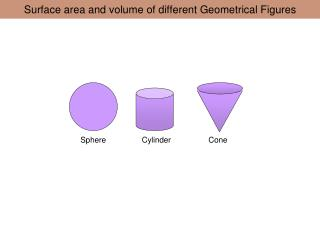# Surface area and volume of different Geometrical Figures - PowerPoint PPT PresentationDownload PresentationSurface area and volume of different Geometrical Figures

Surface area and volume of different Geometrical FiguresDownload Presentation## Surface area and volume of different Geometrical Figures

- - - - - - - - - - - - - - - - - - - - - - - - - - - E N D - - - - - - - - - - - - - - - - - - - - - - - - - - -
##### Presentation Transcript

1. Sphere Cylinder Cone Surface area and volume of different Geometrical Figures

2. Outer Curved Surface area of cylinder r r h Click to animate Activity -: Keep bangles of same radius one over another. It will form a cylinder. Circumference of circle = 2 π r Formation of Cylinder by bangles It is the area covered by the outer surface of a cylinder. Circumference of circle = 2 π r Area covered by cylinder = Surface area of of cylinder = (2 π r) x( h)

3. Total Surface area of a solid cylinder Curved surface circular surfaces = Area of curved surface + area of two circular surfaces =(2 π r) x( h) + 2 π r2 = 2 π r( h+ r)

4. Other method of Finding Surface area of cylinder with the help of paper r h h 2πr Surface area of cylinder = Area of rectangle= 2 πrh

5. Volume of cylinder r h Volume of cylinder = Area of base x vertical height = π r2 xh

6. Cone l = Slant height h Base r

7. Volume of a Cone Click to See the experiment h h Here the vertical height and radius of cylinder & cone are same. r r 3( volume of cone) = volume of cylinder 3( V) = π r2h V = 1/3 π r2h

8. if both cylinder and cone have same height and radius then volume of a cylinder is three times the volume of a cone , Volume = 3V Volume =V

9. Mr. Mohan has only a little jar of juice he wants to distribute it to his three friends. This time he choose the cone shaped glass so that quantity of juice seem to appreciable.

10. Surface area of cone l 2πr l l 2πr Area of a circle having sector (circumference) 2π l = π l 2 Area of circle having circumference 1 = π l 2/ 2 π l So area of sector having sector 2 π r = (π l 2/ 2 π l )x 2 π r = π rl

11. Comparison of Area and volume of different geometrical figures

12. Area and volume of different geometrical figures r r r r/√2 l=2r r

13. Total surface Area and volume of different geometrical figures and nature r r r r l=3r 1.44r 22r So for a given total surface area the volume of sphere is maximum. Generally most of the fruits in the nature are spherical in nature because it enables them to occupy less space but contains big amount of eating material.

14. Think :- Which shape (cone or cylindrical) is better for collecting resin from the tree Click the next

15. 3r r r V= 1/3π r2(3r) V= π r3 Long but Light in weight Small niddle will require to stick it in the tree,so little harm in tree V= π r2 (3r) V= 3 π r3 Long but Heavy in weight Long niddle will require to stick it in the tree,so much harm in tree

16. Bottle Cone shape Cylindrical shape

17. r V1 If we make a cone having radius and height equal to the radius of sphere. Then a water filled cone can fill the sphere in 4 times. r r V=1/3 πr2h If h = r then V=1/3 πr3 V1 = 4V = 4(1/3 πr3) = 4/3 πr3

18. Volume of a Sphere Click to See the experiment r r h=r Here the vertical height and radius of cone are same as radius of sphere. 4( volume of cone) = volume of Sphere 4( 1/3πr2h) = 4( 1/3πr3 ) = V V = 4/3 π r3

19. Thanks U.C. Pandey R.C.Rauthan, G.C.Kandpal